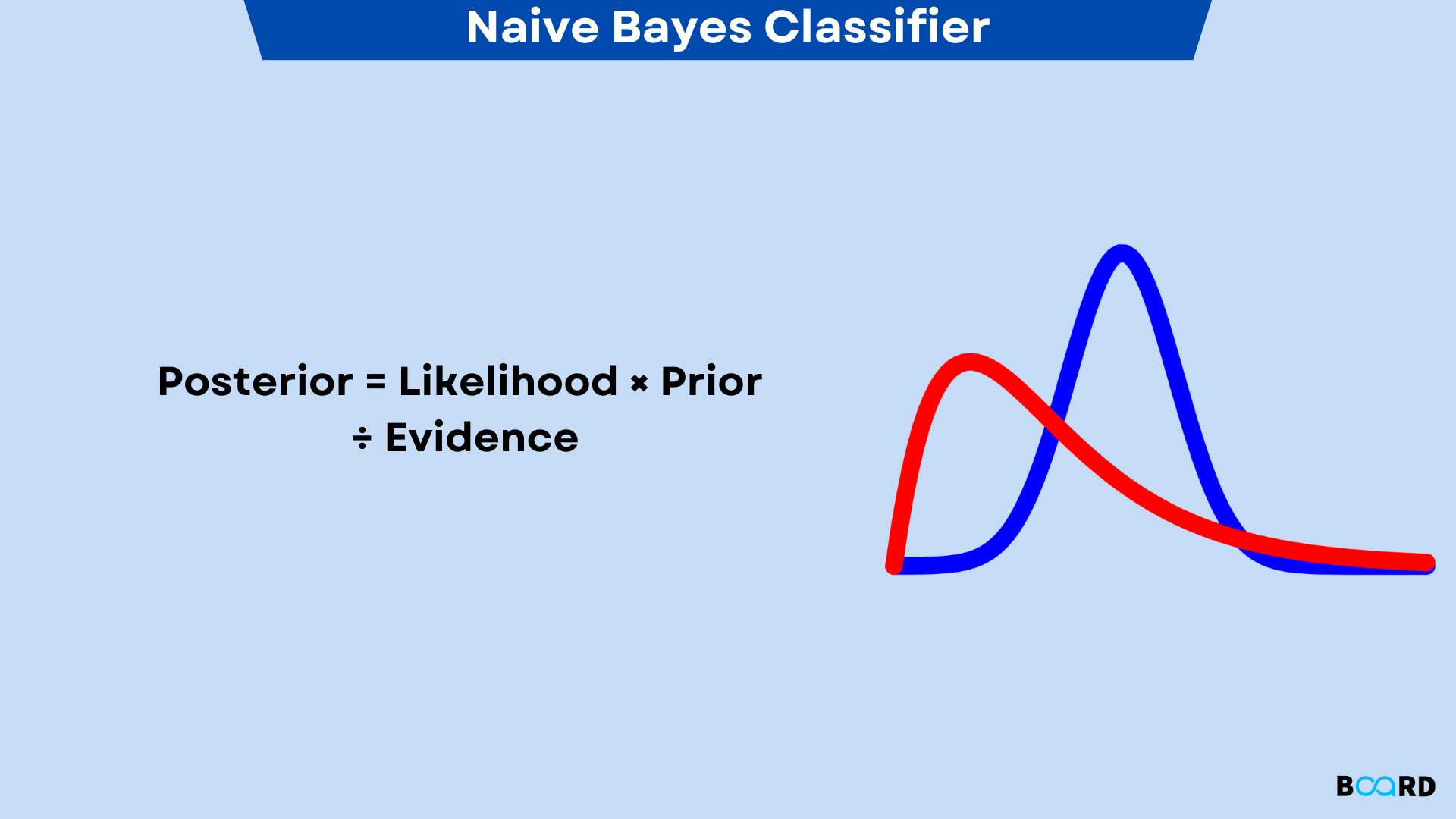# Naive Bayes Classifiers - Overview## Introduction

A probabilistic machine learning model called a Naive Bayes classifier is utilised for classification tasks. The Bayes theorem serves as the foundation of the classifier.

Bayes Theorem:

When B has already happened, we may use the Bayes theorem to calculate the likelihood that A will also occur. Here, A is the hypothesis and B is the supporting evidence. Here, it is assumed that the predictors and features are independent. That is, the presence of one feature does not change the behaviour of another. The term "naive" is a result.

Let's use an illustration to gain a better understanding. Think about the issue of playing golf. The dataset is displayed as follows.

Based on the characteristics of the day, we determine if it is appropriate to play golf. These features are represented by columns, whereas individual items are represented by rows. If we look at the first row of the dataset, we can see that it is not recommended to play golf when it is raining, hot outside, humid, and not windy. As mentioned before, one of our two presumptions is that these predictors are independent. In other words, just because it's hot outside doesn't necessarily mean that it's humid. Another supposition made here is that the influence of each predictor on the result is equal.

Bayes theorem can be rewritten as:

X appears as,

Here, the features are represented by the numbers x 1, x 2,.. x n, which can be translated to outlook, temperature, humidity, and windy. By replacing X and expanding using the chain rule, we obtain,

## Implementation

 iris = load_iris()  # store the feature matrix (X) and response vector (y)X = iris.datay = iris.target  # splitting X and y into training and testing setsfrom sklearn.model_selection import train_test_splitX_train, X_test, y_train, y_test = train_test_split(X, y, test_size=0.4, random_state=1)  # training the model on training setfrom sklearn.naive_bayes import GaussianNBgnb = GaussianNB()gnb.fit(X_train, y_train)  # making predictions on the testing sety_pred = gnb.predict(X_test)  # comparing actual response values (y_test) with predicted response values (y_pred)from sklearn import metricsprint("Gaussian Naive Bayes model accuracy(in %):", metrics.accuracy_score(y_test, y_pred)*100)Gaussian Naive Bayes model accuracy(in %): 95.0

## Naive Bayes Classifier Types:

### Naive Multinomial Bayes

This is mostly used for document classification issues, such as determining whether a document falls under the sports, politics, technology, etc. category. The frequency of the terms included in the document is one of the features/predictors that the classifier uses.

### Bernoulli Naive bayes

Similar to the multinomial naive bayes, the Bernoulli naive bayes uses boolean variables as predictors. The only options for the factors we use to predict the class variable are yes or no, as in whether a word is in the text or not.

### Gaussian Naive Bayes

We assume that the values of the predictors are samples from a gaussian distribution when they take up a continuous value and are not discrete.

## Application

• You can use this algorithm to produce real-time predictions because it is quick and effective.
• Popular for making multi-class predictions, this algorithm. Using this approach, you can quickly determine the probability of many target classes.
• This algorithm is used by email services (like Gmail) to determine if an email is spam or not. For spam filtering, this algorithm works incredibly well.
• It is ideal for performing sentiment analysis due to its assumption of feature independence and effectiveness in addressing multi-class problems. Sentiment analysis is the process of determining if a target group's sentiments are favourable or negative (customers, audience, etc.)
• The Naive Bayes algorithm and collaborative filtering combine to create recommendation systems.

## Summary

The majority of applications for naive Bayes algorithms include sentiment analysis, spam filtering, recommendation systems, etc. Although they are quick and simple to use, their major drawback is the need for independent predictors. The classifier performs worse when the predictors are dependent, which occurs in the majority of real-world situations.This method can accurately estimate the class of a test dataset and operates relatively quickly.It can be used to resolve multi-class prediction issues because it works well with them.If the independence of features is true, the Naive Bayes classifier performs better than other models with less training data.

The Naive Bayes algorithm performs far better than numerical variables when you have categorical input variables.It can be utilised for multi-class and binary classifications.It functions well in predictions for several classes.